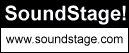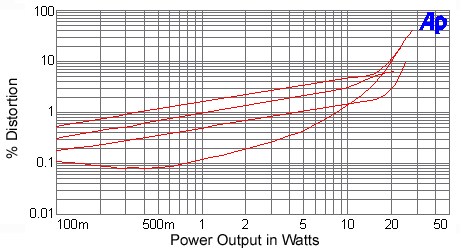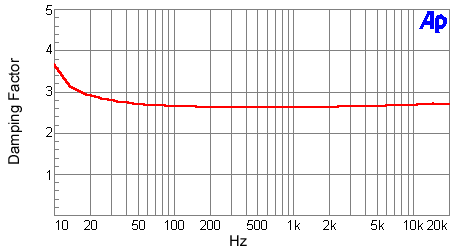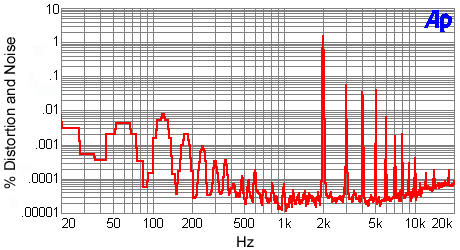Home Audio
Equipment Measurements

June 2003

Wyetech Labs Topaz 572B Stereo Amplifier: Measurements

All amplifier measurements are performed independently by BHK Labs. Please click to learn more about how we test amplifiers there. All measurement data and graphical information displayed below are the property of SoundStage! and Schneider Publishing Inc. Reproduction in any format is not permitted.

• Measurements were made with 120V AC line voltage.
• Power output and distortion plotted with both channels driven (worse of two channels shown).
• Gain: 20.8x, 26.4dB.
• Output noise, 8-ohm load, unbalanced input, 1k-ohm input termination: wideband 1.0mV, -69.0dBW; A weighted 0.15mV, -85.5dBW.
• AC line current draw in "operate" mode: 3.6A.
• AC line current draw in "standby" mode: 0.89A.
• Output impedance at 50Hz: 2.86 ohms.
• This amplifier does not invert polarity.
 Measurements Summary

Power output with 1kHz test signal

• 8-ohm load at 1% THD: 4.5W
• 8-ohm load at 10% THD: 25W

• 4-ohm load at 1% THD: 1W
• 4-ohm load at 10% THD: 30W

• 16-ohm load at 1% THD: 8.5W
• 16-ohm load at 10% THD: 20W

General

The Wyetech Labs Topaz 572B SET (single-ended triode) amplifier measured here is typical of a design utilizing the Sovtek SV 572 10 output tube. This tube replaces the 211 used in the earlier version of this amplifier and allows for higher class-A output power.

Frequency response, as seen in Chart 1, rolls off at both ends of the audio range, and there is some pronounced ultrasonic aberrations likely due to the characteristics of the output transformer. The low-frequency response holds up down to 20Hz -- better than most of the low- or no-feedback SET designs. Output impedance is moderate, giving an approximate plus-or-minus 2dB frequency-response variation on the NHT dummy speaker load. Total harmonic distortion plus noise as a function of power output and load on the 8-ohm output for a test frequency of 1kHz is plotted in Chart 2. Also shown in this chart is the SMPTE IM distortion for an 8-ohm load on the 8-ohm output. It shows that the amount of distortion at the 1kHz test frequency is reasonably low, at less than 2% up to 10W output for the 8-ohm load (and measures similarly for a 4-ohm load on the 4-ohm output). Raising the load to 16 ohms increases the distortion somewhat, whereas decreasing the load to 4 ohms reduces the amount of distortion up to about 1W. This is because the dominant second harmonic is partially canceled by this operating condition. Total harmonic distortion plus noise as a function of frequency at several power levels is plotted in Chart 3. Amount of distortion over the main midrange energy band is less than 2% for power outputs of 10W or less. Admirable is the relatively low amount of distortion increase at the higher frequencies. However, distortion does rise considerably at the low end of the audio range. Damping factor vs. frequency, shown in Chart 4, is a modest 2.6 over the mid range of frequencies, and actually increases a bit at the frequency extremes. As is usual, on the 4-ohm output, the damping factor was about double. In the spectral plot of distortion plus noise for a 10W 1kHz signal into an 8-ohm load on the 8-ohm output, the signal distortion components are dominated by the second harmonic with the third, fourth, and fifth harmonics being about equal at 1/20 of the amount of the second harmonic. The amount of AC line hum components adding up to about 1mV would likely be audible on speakers with efficiencies of 90dB or more.

 Chart 1 - Frequency Response of Output Voltage as a Function of Output LoadingMagenta line: open circuit

 Chart 2 - Distortion as a Function of Power Output and Output Loading(line up at 2W to determine lines)
Top line: 8-ohm SMPTE IM
Second line: 4-ohm THD+N
Third line: 8-ohm THD+N
Bottom line: 16-ohm THD+N

 Chart 3 - Distortion as a Function of Power Output and FrequencyCyan line: 15W
Blue line: 10W
Magenta line: 5W
Red line: 1W

 Chart 4 - Damping Factor as a Function of FrequencyDamping factor = output impedance divided into 8

 Chart 5 - Distortion and Noise Spectrum1kHz signal at 10W into an 8-ohm loadAll Contents